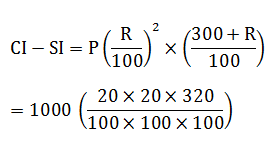# SSC Quantitative Aptitude Questions (Day-50)

Dear Aspirants, you can find the Quantitative Aptitude questions with detailed explanations for the SSC exams. Nowadays the competitive level of the exam has been increasing consistently. Due to the great demand for the government job, the level of the toughness reached greater. Candidates have to enhance the preparation process in order to drive in the right path. It doesn’t need to clear the prescribed cutoff. You must have to score good marks more than the cut off marks to get into the final provisional list. Here we have updating the Quantitative Aptitude questions with detailed explanations on a daily basis. You can practice with us and measure your level of preparation. According to that you can sculpt yourself in a proper way. SSC aspirants kindly make use of it and grab your success in your career.

Start Quiz

1) In what ratio wheat at Rs 32/kg should be mixed with wheat at Rs 24/kg so that on selling the mixture at Rs 39/kg there is a profit of 30%?

(a) 3 : 1

(b) 2 : 3

(c) 1 : 4

(d) 2 : 5

2) The average runs scored by a batsman in 7 matches is 53 and in other 9 matches the average is 33. What is the average runs scored by the batsman in16 matches?

(a) 41.75

(b) 44.25

(c) 47

(d) 49.175

3) The average marks obtained by 150 students in an examination is 40. If the average marks of passed students is 60 and that of the failed students is20, then what is the number of students who passed the examination?

(a) 25

(b) 50

(c) 75

(d) 100

4) A trader sold an article at a gain of 20%. Had he purchased it for 40% more and sold for Rs 24 less, then he would have incurred a loss of 20%. Whatis the cost price (in Rs) of the article?

(a) 150

(b) 300

(c) 450

(d) 600

5) The cost price of a chair is 25% less than the cost price of a table. If a man sells 5 chairs at the cost price of 7 tables, then what is his profitpercentage?

(a) 33.33

(b) 45

(c) 86.66

(d) 96

6) If the radius of a circle is decreased by 10%, then what will be the percentage decrease in the area of circle?

(a) 19

(b) 20

(c) 21

(d) 23

7) If A is 50% more than B. B is how much percent less than A?

(a) 50

(b) 100

(c) 66.66

(d) 33.33

8) A car travels 20% slower than a train. Both starts from point A at the same time and reach point B 240 km away at the same time. On the way the traintakes 48 minutes for stopping at the stations. What is the speed (in km/hr) of the car?

(a) 80

(b) 100

(c) 120

(d) 60

9) A scooter runs at a speed of 49 km/hr after repairing and runs at 42 km/hr before repairing. It covers a certain distance in 7 hours after repairing. Howmuch time will it take to cover twice of the distance before repairing?

8 hours 10 mins

12 hours 20 mins

16 hours 20 mins

18 hours 30 mins

10) What is the difference (in Rs) between compound interest and simple interest for 3 years on a principal of Rs 1000 at the rate of 20% per annum?

(a) 64

(b) 120

(c) 128

(d) 136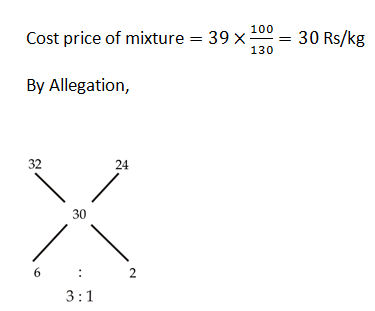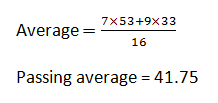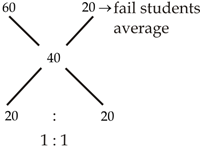2R → 150

1R = 75

OR

Let number of students who passed the exam = x

Now,

150 × 40 = 60x + 20(150 – x)

6000 = 60x + 3000 – 20x

3000 = 40x

x = 75

Let CP = 100x. then SP = 120x

New CP =140x

New SP = 120x – 24Rs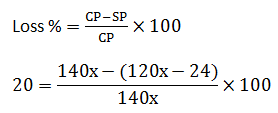On solving

then, x = 3

CP = 100 × 3 = 300 Rs

CP of Chair = 3x

CP of table = 4x

CP of 5 chairs = 15x

SP of 5 chairs= 28x

Profit percentage = 13x/15x × 100

= 86.66

Area ; 100 → 81

% decrease =19/100 × 100

= 19%

OR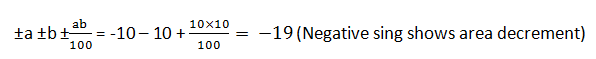A : B = 3 : 2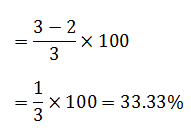Ratio of speed of train to car = 5 : 4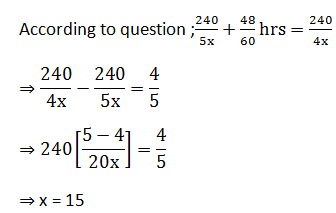Speed of car = 4x = 4 × 15 = 60 km/hr

Distance covers after repairing = 49 × 7 = 343 km

Time takes  Before reparing to cover twice distance = (343 × 2) / 42

= 49/3 hr

= 16 hr 20 minutes